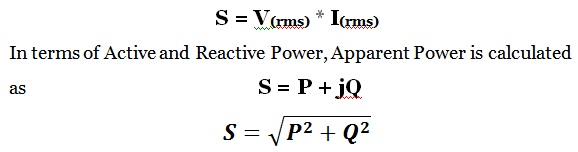# Electrical Power- Active, Reactive and Apparent Power

0
648

## Active Power (kW)

#### In the case of DC circuit or in pure resistive AC circuit, active power is calculated as the product of voltage and current in the circuit. Whereas in the case of AC circuit it is calculated as:## Reactive Power (kVAR)

#### In the case of the DC circuits, there are no concepts of Reactive Power. Whereas for the AC circuit it is calculated as## Apparent Power (kVA):

#### In the case of the DC circuit, it is the total power of the circuit. Whereas for the AC circuit it is calculated as :